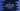# C program to calculate the total number of lines in a file### C program to calculate the total number of lines in a file :

In this tutorial, we will learn how to find the total number of lines in a file using C programming language. To run the program, just change the path of the file to your own file path. Before going in details how this program works, let’s take a look into the program first :

### C program to find the total number of lines in a file :

``````#include

int main()
{
//1
FILE *file;

//2
int totalLinesCount = 0;
char currentCharacter;

//3
file = fopen("C://MyFile.txt","r");

//4
if(file == NULL){
printf("The file doesn't exist ! Please check again .");
return 0;
}

//5
while((currentCharacter = fgetc(file)) !=  EOF){
//6
if(currentCharacter == '\n'){
totalLinesCount ++;
}
}

//7
fclose(file);

//8
totalLinesCount ++;

//9
printf("Total number of lines are : %d\n",totalLinesCount);

return 0;
}``````

### Explanation :

The commented numbers in the above program denotes the below step number :

1. Create one file pointer variable.
2. Create one variable totalLinesCount to store the total number of lines. Also, create one more variable currentCharacter to store the current reading character of that file.
3. Open the file in read mode (“r”) and store it in file pointer variable file.
4. If the variable file is NULL, means the given file is not available. Print one error message and return.
5. Now, run one while loop to read the file character by character. This loop will complete when the file ends.
6. For each character, we are checking if the character is newline character or \n. If any newline character found , increment the variable totalLinesCount.
7. After the loop is completed, close the file. Always remember to close the file if you have opened it.
8. We are incrementing the total count one more time because the last line will not contain any newline character.
9. Finally, print out the total number of lines we have calculated.

### Example Output :

For the following file :

``````This is a line
This is second line
Third line
Fourth
Fifth
Last line``````

It will give 6 as output